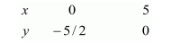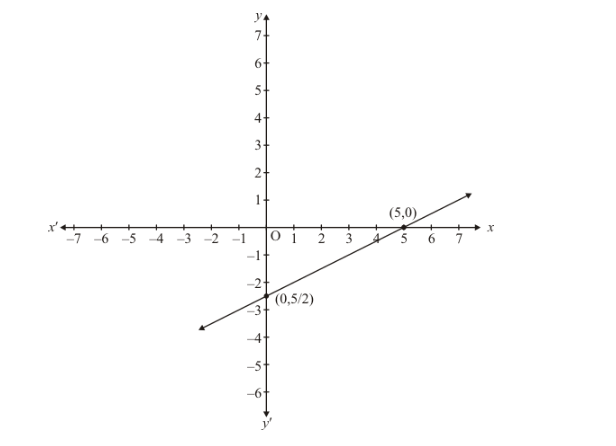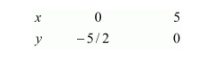# Show graphically that each one of the following systems of equations has infinitely many solutions`
Question:

Show graphically that each one of the following systems of equations has infinitely many solutions:

$x-2 y=5$

$3 x-6 y=15$

Solution:

The given equations are

$x-2 y=5$ ...(i)

$3 x-6 y=15$...(ii)

Putting $x=0$ in equation $(i)$, we get:

$\Rightarrow 0-2 y=5$

$\Rightarrow y=-5 / 2$

$x=0, \quad y=-5 / 2$

Putting $y=0$ in equations $(i)$ we get:

$\Rightarrow x-2 \times 0=5$

$\Rightarrow x=5$

$x=5, \quad y=0$

Use the following table to draw the graph.Draw the graph by plotting the two points $A(0,-5 / 2)$ and $B(5,0)$ from tableGraph of the equation….$3 x-6 y=15$..(ii)

Putting $x=0$ in equations $(i i)$ we get:

$\Rightarrow 3 x-6 \times 0=15$

$\Rightarrow x=5$

$x=5, \quad y=0$

Use the following table to draw the graph.Draw the graph by plotting the two points $C(0,-5 / 2)$ and $D(5,0)$ from table.

Thus the graph of the two equations coincide

Consequently, every solution of one equation is a solution of the other.

Hence the equations have infinitely many solutions.